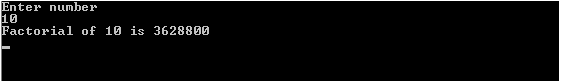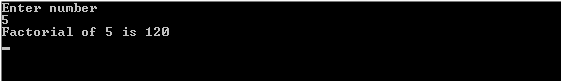# Factorial Program in C

Factorial program in c using function and recursion with the output is given below.

The factorial of a non negative integer, n, is the product of all positive integers less than or equal to n. It is denoted by n!. Also, the value of 0! is 1.

For example,
3!=3*2*1=6

Recursively, factorial can be defined as,
n! = 1 when n=0
n! = (n-1)! * n when n>0

## Factorial Program in C Without Using Function

```// Factorial program in c
#include<stdio.h>
main()
{
int x,fact=1,i;
printf("Enter numbern");
scanf("%d",&x);
for(i=1;i<=x;i++)
{
fact=fact*i;
}
printf("Factorial of %d is %dn",x,fact);
getch();
return 0;
}

```

### Output## Factorial Program in C Using Function

```// Factorial program in c using function
#include<stdio.h>
main()
{
int x;
printf("Enter numbern");
scanf("%d",&x);
printf("Factorial of %d is %dn",x,fact(x));
getch();
return 0;
}
int fact(int x)
{
int fact=1,i;
for(i=1; i<=x; i++)
fact*=i;
return fact;
}

```

### Output## Factorial Program in C Using Recursion

```// Factorial program in c using recursion
#include<stdio.h>
main()
{
int x;
printf("Enter numbern");
scanf("%d",&x);
printf("Factorial of %d is %dn",x,fact(x));
getch();
return 0;
}
int fact(int x)
{
if(x==0)
return 1;
return (x * fact(x-1));
}

```

Please comment with your real name using good manners.

This site uses Akismet to reduce spam. Learn how your comment data is processed.